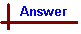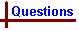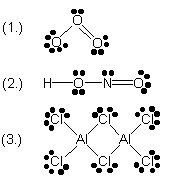Shortcut to Questions

Q: 1 2 3 4 5 6 7 8 9 10 11

1

Potassium and liquid bromine interact violently to produce a white ionic solid, potassium bromide.

(1.) List the equations for the reactions required to produce K+(g) and Br-(g) from the solid potassium and the liquid bromine.

(2.) ΔH for the sum of this series of reactions is +277 kJ per mole of potassium. Does this imply that the reaction is likely or unlikely to occur?

(3.) Write the equation for the reaction which produces potassium bromide from K+(g) and Br-(g).

(4.) Experimentally, the overall reaction between the elements occurs exothermically. Is ΔH for the final reaction positive or negative?

(5.) What is the name of the energy term for the final reaction described in (3.) Define the term named in (3.).2

What are the two extremes for the distribution of a pair of electrons between two atoms?3

Give examples of stable molecules in which the central atom does not have an octet of electrons (ie where the octet rule is not satisfied).4

(1.) Diamond melts at 3773 K, while iodine melts at 387 K. Explain this in terms of their crystal structures.

(2.) Give one further example of each of the two kinds of crystal structure illustrated in (1.).5

Classify the dipole moments of the following molecules as either "zero" or "nonzero". For online tutorial help with this topic, see the chemcal module "Electronegativity and Polar Molecules"

(1.) hydrogen iodide

(2.) carbon dioxide

(3.) ethanol

(4.)
1-chloro-2-butyne (Cl-CH2C≡CH3)

(5.) nitrogen dioxide6

Complete the following table.
 Species Hybridisation of central atom Expected bond angle Number(s) and type(s) of bonds around central atom CCl4 sp3 109.5° sigma bonds CO2 sp 2 sigma and 2 pi bonds HCN 180° Cl2CO approx 120° NH4+ BCl3 120° SF6 sp3d2 BeCl2 2 sigma bonds7 (1) Complete the following table. For each of the last two columns, chose one of the following shapes: linear, angular (bent), trigonal planar, pyramidal, tetrahedral or none of these. For online tutorial help with this topic, see the chemcal modules "Molecular Shape and Structure" and "Applying VSEPR Theory"

 Molecule Hybridisation of the central atom Arrangement of valence shell electron pairs Shape of molecule NH3 sp3 tetrahedral trigonal pyramidal BF3 H2O CO2 GeCl2

(2) Which of the five molecules in the table has the smallest angle between the bonds joining the central atom and the remaining atoms?

(3) List all molecules in the table which are non-polar - i.e. have a zero dipole moment.8

Arrange the following entities in order of increasing bond angle between a non-central atom, the central atom and a non-central atom: carbonate ion, carbon tetrachloride molecule, water molecule, hydrogen cyanide molecule.9

Write a suitable electron dot (Lewis) formula for each of the following molecules. Show all valence electrons including lone pairs. For online tutorial help with this topic, see the chemcal module "Covalent Bonding"

(1.) ozone (O3)

(2.) nitrous acid (HNO2)

(3.) dialuminium hexachloride (Al2Cl6)10

For each of the entities listed write the structural formula (showing lone pairs) and give the hybrid state of the selected atom(s) shown in parenthesis beside it.

(1.) Phosphorus pentachloride molecule (P)

(2.) Iodine pentafluoride molecule (I)

(3.) Nitrous acid molecule (N)

(4.) Acetic (ethanoic) acid molecule (C1 and C2)

(5.) Hydrogen peroxide molecule (O)

(6.) Hydrogen cyanide molecule (C)

(7.) Tetrafluoroborate ion (B)

(8.) Sulfur hexafluoride molecule (S)

(9.) Water molecule (O)

(10.) Dicyanoargentate ion (Ag)

(11.) Mercury(II) bromide molecule (Hg)

(12.) Formic (methanoic) acid molecule (C)1

(1.) Solid potassium vaporising and then ionising:

K(s) K(g)

K(g)K+(g) + e-

Liquid bromine (Br2(l)) vaporising, and then ionising:

½Br2(l) → ½Br2(g)

½Br2(g) Br(g)

Br(g) + e-Br-(g)

(2.) The positive enthalpy sum for this set of reactions indicates they require energy to proceed (ie. they are endothermic). The sign of the enthalpy change for a reaction is not the predictor of spontaneity for that reaction. Instead it is the sign of the free energy change, ΔG , which predicts the spontaneous direction of a reaction. However, if as in this case ΔH is large and positive, it is less likely that these reactions as written will occur spontaneously.

(3.) Reaction:

K+(g) + Br-(g)KBr(s)

A solid lattice of K+ and Br- ions forms.

(4.) An exothermic reaction indicates a negative ΔH value (ie heat is released)

(5.) The energy released in this step is the lattice energy which is defined as the energy required for the dissociation of one mole of a crystal into its constituent gaseous ions. The process can be represented by the general equation MnXy(s) → My+(g) + Xn-(g). When defined in this way, energy is absorbed so the sign of ΔH for this process is positive. From Part (4), the energy released when the same gas-phase ions combine to form the solid crystal is identical in magnitude but the sign of ΔH is negative.2

The bonding electrons between 2 atoms can be equally shared between the two bonding atoms on one extreme (ie a non-polar covalent bond), or completely localised and fixed around only one atom (ie an ionic bond). However, most bonds actually involve a degree of sharing somewhere between these two extremes. ie all ionic bonds involve some degree of sharing, and the only non-polar bonds in which electrons are completely evenly shared are those between identical atoms (eg H-H).3

Some examples include:

BeCl2 (4 bonding electrons)

BF3 (6 bonding electrons)

PCl5 (10 bonding electrons)

SF6 (12 bonding electrons)

Also, many transition metal ions do not satisfy the octet rule.

In fact, the octet rule is only really applicable to the elements carbon fluorine in Period 2. In subsequent periods of the table, the availability of d-orbitals allows atoms to hold more than 8 electrons in their valence level. The term "expansion of the octet" is sometimes used to describe the situation found in compounds such as PCl5 and SF6 above.4

(1.) Diamond is an example of a network covalent bonded solid. The strong covalent bonding, which extends throughout the entire crystal, typically results in solids with very high melting points.

Iodine is an example of a molecular covalent bonded solid, in which covalent bonds exist between the two atoms of the I2 molecule. However, between molecules there exists only weak dispersion forces. It is these weak dispersion forces which are overcome when an iodine crystal is melted to release individual I2 molecules (note - not I atoms as this would require the strong covalent I-I bonds to be broken), so the melting point is relatively low.

(2.) Other examples of network covalent bonding are silicon carbide (SiC), silicon dioxide (quartz, SiO2), and the network covalent crystalline form of boron nitride ("borazon", BN). Other examples of molecular covalent bonding are solid CO2 and solid O2 and most organic compounds which have low melting points.5

The diopole moment is a measure of the overall polarity of a molecule. A dipole moment of zero indicates no molecular polarity, whilst increasing non-zero values indicate increasing molecular polarity. There is a number of factors which influence the polarity of a molecule, including the electronegativities of atoms present and the shape of the molecule. (Note that determining the molecular shape is very important, since the presence of molecular symmetry can radically change the way individual polar bonds contribute to the overall molecular polarity.

(1.) hydrogen iodide, H-I. The electronegativity of hydrogen is 2.1 (on the unitless Pauling scale), and that of iodine is 2.5. Therefore iodine is more electronegative than hydrogen and we would expect the H-I bond to be polar (ie the bonding electrons spend somewhat more time near the more electronegative iodine atom than the less electronegative hydrogen atom). Since the molecule is composed only of the H-I bond we can say that there is an overall molecular polarity, and therefore the dipole moment will be non-zero.

(2.) carbon dioxide, CO2. The electronegativity of oxygen is greater than that of carbon, so the C=O bond is polar. Now the shape of the molecule must be determined. Carbon has four outer electrons, and is involved in two C=O double bonds. Therefore the molecular shape is linear (ie 180° O=C=O bond angle). It is now evident that there is no net molecular polarity since the two polar C=O bonds points in opposite directions to each other, and hence their contributions cancel out. The dipole moment of CO2 is therefore zero.

In contrast, SO2 (a bent, rather than linear molecule) exhibits a net molecular dipole since the polar S=O bonds are not oriented directly opposite each other and their bond dipoles do not completely cancel.

(3.) ethanol, CH3CH2OH. Note the presence of an oxygen atom which is considerably more electronegative than either carbon or hydrogen. The oxygen atom is located at one end of the molecule, so electron density will be higher at this end. The shape of the molecule is angular at the C-O-H bond. Therefore the molecule posesses a dipole moment.

(4.) 1-chloro-2-butyne, CH3CC-CH2Cl. This molecule contains a polar C-Cl bond located at one end of the molecule. There is no symmetry which cancels the polar effect out, so there is an overall molecular symmetry, and the overall molecular dipole moment is non-zero.

(5.) nitrogen doxide, NO2. Nitrogen dioxide contains a polar N-O bond and a polar N=O bond. Nitrogen posesses 5 bonding elecrons, one of which remains unbonded in NO2 (ie a lone electron). Lone electrons exert a repulsive effect on surrounding bonds in much the same way as lone pairs. As a result, the O-N=O bond angle is less than 180°, and the polar N-O and N=O bonds create a net non-zero molecular dipole moment.6

CO2. The central C atom exhibits sp hybridisation, so the expected bond angle is 180° (ie linear).

HCN. The bond angle is 180°, so the hybridisation of the central atom is sp. The C-H bond is a single sigma bond, whilst the triple CN bond is composed of one sigma and 2 pi bonds. This gives a total of 2 sigma bonds and 2 pi bonds.

Cl2CO. The bond angle is 120°, with three atoms bonding to the central C, so the hybridisation is sp2. There are two single C-Cl bonds and one double C=O bond, giving a total of 3 sigma bonds and 1 pi bond.

NH4+. The ammonium ion is a tetrahedral ion (same shape as CCl4 or CH4). There are four H atoms bonded to the central N and no lone pairs, so the hybridisation of the N atom must be sp3. The expected bond angle in a tetrahedral shape is 109.5°. All four bonds are single N-H bonds, so there are 4 sigma bonds around the N atom. [Note that the ammonium ion is formed from an ammonia molecule, NH3 , to which an H+ ion is bonded on the central N atom using the lone pair of electrons on N atom. However, once formed, all four N-H bonds are identical.]

BCl3. There are three atoms bonded to the central B atom and no lone electrons on that atom, so the hybridisation is sp2. All three of these bonds are single B-Cl bonds, so there are 3 sigma bonds present.

SF6. There are six atoms bonded to the central S atom and no lone electrons left, resulting in an octahedral molecular shape with the F-S-F bond angles equal to 90°. Each of these 6 bonds are single F-S bonds, so there are 6 sigma bonds around the central S.

BeCl2. Be has no lone pairs in BeCl2, so the shape will be linear with a 180° Cl-Be-Cl angle. The hybridisation of the Be atom is sp.7

(1.) The hybridisation of the central atom is determined by considering the arrangement of the valence shell - note that molecular shape does not always determine the central atom's hybridisation, since lone pairs will influence the hybridisation of the central atom as well as bonding pairs because they also require an orbital. In the example given, NH3, the valence shell arrangement is tetrahedral, since there are three N-H bonds and a lone pair while the shape is trigonal pyramidal as the lone pair is not counted as part of the shape description. In summary:

Molecular shape describes the arrangement of atoms around the central atom. In the case of NH3 the arrangement of the three N-H bonds is trigonal pyramidal (the N lone pair is non-bonding, and hence not included.)

Valence shell arrangement describes the arrangement of all electron pairs (bonding pairs and non-bonding lone pairs) around the central atom. In the case of NH3 the presence of the N lone pair is included along with the 3 bonding pairs, so the arrangement of the four electron pairs is tetrahedral.

The atom's hybridisation is determined from the valence shell arrangement. This leads to the assignment of sp3 hybridisation of N, based on the tetrahedral valence shell arrangement.

BF3. There are 3 B-F bonds present. Boron is a group 13 element and posesses 3 outer shell valence electrons. All of these 3 electrons are involved in bonding, so there are no non-bonding electrons (ie no lone electrons or lone pairs) present. Therefore the valence shell arrangement and molecular shape are both trigonal planar. This leads to an sp2 assignment for the central boron atom.

H2O. There are 2 O-H bonds present. Oxygen is a group 16 element, so it posesses 6 outer shell valence electrons. Two of these electrons are bonding with hydrogen (1 in each of the O-H bonds), which leaves 4 electrons not involved in any bonds. These non-bonding electrons are therefore present as 2 lone pairs on the central oxygen atom. This leads to a total of 4 electron pairs present on the central oxygen - 2 bonding pairs (one pair in each O-H bond), and 2 non-bonding pairs (two lone pairs on the O atom). Four electron pairs will arange themselves in a tetrahedral configuration, so the valence shell arrangement is tetrahedral. We can now assign the hybridisation of the central O as sp3, and the molecular shape as bent or angular (the two O lone pairs are ignored, and only the two bonding O-H bonds are considered - they form a bent molecular shape

BeBr2. There are 2 Be-Br bonds present. Beryllium, a group 2 element, posesses 2 outer shell valence electrons. Both of these are involved in bonding, so there are no non-bonding outer electrons present in the central Be atom. Therefore the valence shell arrangement and molecular shape are both linear. This leads to an sp hybridisation of the central Be atom.

CO2. Carbon is a group 14 element, and posesses 4 outer shell valence electrons. All four of these electrons are involved in bonding to the O atoms, each of which is joined to the C atom by one sigma and one pi bond. Thus there are no lone electrons on the C atom and the arrangement of the two double bonds about the central C atom is therefore linear. The C atom is sp hybridised.

(2.) Both bonding and non-bonding electrons exert a repulsive effect on each other. If all bond-bond repulsions within a molecule are equal, the bond angles will be exactly the "ideal" value - for example the bond angle in a perfect tetrahedron is 109.5°. Slight differences in repulsive strength result in bond angles which deviate somewhat from this value. For similar molecules of a given valene shell arrangement the deviation from the ideal bond angle will be:

Lone pair - lone pair > lone pair - bonding pair > bonding pair - bonding pair.

ie. Lone pairs exhibit a higher repulsive force than bonding pairs.

Of the molecules listed in the table, tetrahedral valence shell arrangements (H2O, NH3) will have smaller bond angles (ideal bond angle 109.5°) than either the trigonal planar (BF3, ideal bond angle 120°) or linear (BeBr2 and CO2 ideal bond angle 180°) arrangements.

There is one N lone pair present in the NH3 molecule, and two O lone pairs present in H2O. We would expect the repulsive effect of two lone pairs to be more marked than that of one, thus H2O should exhibit the smallest bond angle. (This is confirmed experimentally - the measured H-N-H bond angle in NH3 is 107.3°, whilst the H-O-H bond in H2O is 104.5°.)

(3.) All the molecules in the table contain polar bonds (ie bonds between elements of differing electronegativities). The molecular shape will therefore determine the overall molecular polarity, and whether a molecule has a dipole moment.

NH3 is trigonal pyramidal, so the molecule is polar (ie has a net dipole moment).

BF3 is trigonal planar, so the effect of the three polar B-F bonds cancels out, and the molecule is therefore non-polar (ie has no dipole moment)

H2O is bent, so there is an overall molecular polarity, and the dipole moment will be non-zero.

BeBr2 is linear, so the effect of the two polar Be-Br bonds cancels, and the dipole moment is zero.

CO2 is linear, so the effect of the two polar C=O bonds cancels, and the dipole moment is zero.8

First we assign valence shell arrangements:

CO32-: trigonal planar

CCl4: tetrahedral

H2O: tetrahedral

HCN: linear

CCl4 and H2O both have tetrahedral valence shell arrangements, but the H-O-H bond is subject to distortion by the two O lone pairs, resulting in a smaller bond angle than that of CCl4.

Therefore the molecules can be arranged in order of increasing bond angle:

H2O < CCl4 < CO32- < HCN910